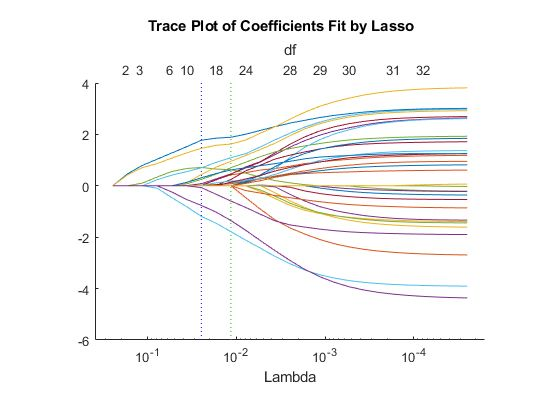# Avoiding Pitfalls Such as Overfitting

Overfitting means your model is so closely aligned to training data sets that it does not know how to respond to new situations. This chapter discusses:

• Why overfitting is difficult to avoid
• How to correct overfitting
• How to prevent overfitting

## Why overfitting is difficult to avoid

One of the reasons overfitting is difficult to avoid is that it is often the result of insufficient training data. The person responsible for the model may not be the same person responsible for gathering the data, who may not even realize how much data is needed to supply training and testing phases.

An overfit model returns very few errors, which makes it look attractive at first glance. Unfortunately, there are too many parameters in the model in relation to the underlying system. The training algorithm tunes these parameters to minimize the loss function, but this results in the model being overfit to the training data, rather than the desired behavior of generalizing the underlying trends. When large amounts of new data are introduced to the network, the algorithm cannot cope, and large errors begin to surface.

Ideally, your machine learning model should be as simple as possible and accurate enough to produce meaningful results. The more complex your model, the more prone it will be to overfitting.

## How do you avoid overfitting?

The best way to avoid overfitting is by making sure you are using enough training data. Conventional wisdom says you need a minimum of 10,000 data points to test and train a model, but that number depends greatly on the type of task you’re performing (naive Bayes and k-nearest neighbor both require far more sample points). That data also needs to accurately reflect the complexity and diversity of the data the model will be expected to work with.## How to correct overfitting

If you’ve already started working and think your model is overfitting the data, you can try correcting it using regularization. Regularization penalizes large parameters to help keep the model from relying too heavily on individual data points and becoming too rigid. The objective function changes so that it becomes Error $$+λf(Ɵ)$$, where $$f(Ɵ)$$ grows larger as the components of $$Ɵ$$ grow larger and $$λ$$ represents the strength of the regularization.

The value you pick for $$λ$$ decides how much you want to protect against overfitting. If $$λ=0$$, you aren’t looking to correct for overfitting at all. On the other hand, if the value for $$λ$$ is too large, then your model will keep $$Ɵ$$ as small as possible (over having a model that performs well on your training set). Finding the best value for $$λ$$ can take some time to get right.

## How to prevent overfitting

An important step when working with machine learning is checking the performance of your model. One method of assessing a machine learning algorithm’s performance is cross-validation. This technique has the algorithm make predictions using data not used during the training stage. Cross-validation partitions a data set and uses a subset to train the algorithm and the remaining data for testing. Because cross-validation does not use all the data to build a model, it is a commonly used method to prevent overfitting during training.

Each round of cross-validation involves randomly partitioning the original data set into a training set and a testing set. The training set is then used to train a supervised learning algorithm, and the testing set is used to evaluate its performance. This process is repeated several times, and the average cross-validation error is used as a performance indicator.

## Common cross-validation techniques:

Partitions data into k randomly chosen subsets (or folds) of roughly equal size. One subset is used to validate the model trained using the remaining subsets. This process is repeated k times, such that each subset is used exactly once for validation.

Partitions data into exactly two subsets of specified ratio for training and validation.

Partitions data using the k-fold approach, where k is equal to the total number of observations in the data. Also known as leave-one-out cross-validation.

Performs Monte Carlo repetitions of randomly partitioned data and aggregates results over all the runs.

Partitions data such that both training and test sets have roughly the same class proportions in the response or target.

Does not partition the data; uses the training data for validation. Often produces overly optimistic estimates for performance and must be avoided if there is sufficient data.

In the equation Error + λf(Ɵ), what does λ represent?

Nice try!

Regularization penalizes large parameters to help keep the model from relying too heavily on individual data points and becoming too rigid. The objective function changes so it becomes Error $$+λf(Ɵ)$$, where $$f(Ɵ)$$ grows larger as the components of $$Ɵ$$ grow larger and $$λ$$ represents the strength of the regularization. The correct answer is the strength of the regularization.

Nice try!

Regularization penalizes large parameters to help keep the model from relying too heavily on individual data points and becoming too rigid. The objective function changes so it becomes Error $$+λf(Ɵ)$$, where $$f(Ɵ)$$ grows larger as the components of $$Ɵ$$ grow larger and $$λ$$ represents the strength of the regularization. The correct answer is the strength of the regularization.

YOU’RE RIGHT!

Regularization penalizes large parameters to help keep the model from relying too heavily on individual data points and becoming too rigid. The objective function changes so it becomes Error $$+λf(Ɵ)$$, where $$f(Ɵ)$$ grows larger as the components of $$Ɵ$$ grow larger and $$λ$$ represents the strength of the regularization.